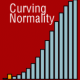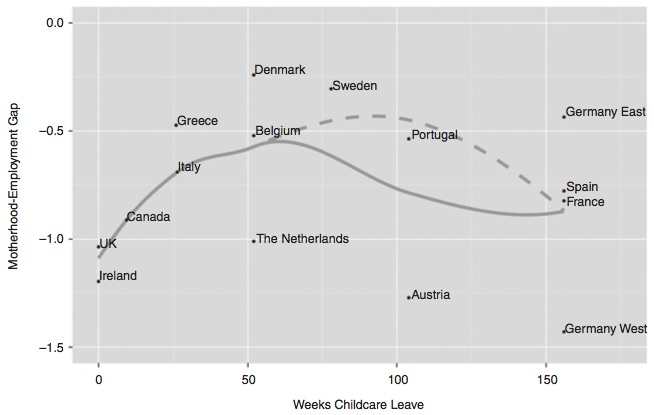18 Apr# A novel method for modelling interaction between categorical variables

We have been developing weighted effect coding in an ongoing series of publications (hint: a publication in the R Journal will follow). To include nominal and ordinal variables as predictors in regression models, their categories first have to be transformed into so-called ‘dummy variables’. There are many transformations available, and popular is ‘dummy coding’ in which the estimates represent deviations from a preselected ‘reference category’.

To avoid choosing a reference category, weighted effect coding provides estimates representing deviations from the sample mean. This is particularly useful when the data are unbalanced (i.e., categories holding different numbers of observation). The basics of this technique, with applications in R, were detailed here.

In a new publication, available open access,, we show that weighted effect coding can also be applied to regression models with interaction effects (also commonly referred to as moderation). The weighted effect coded interactions represent the additional effects over and above the main effects obtained from the model without these interactions.

To apply the procedures introduced in these papers, called weighted effect coding, procedures are made available for R, SPSS, and Stata. For R, we created the ‘wec’ package which can be installed by typing:

install.packages(“wec”)

# References (Open Access!)

Grotenhuis, M., Ben Pelzer, Eisinga, R., Nieuwenhuis, R., Schmidt-Catran, A., & Konig, R. (2017). A novel method for modelling interaction between categorical variables. International Journal of Public Health, 62(3), 427–431. http://link.springer.com/article/10.1007/s00038-016-0902-0

Grotenhuis, M., Ben Pelzer, Eisinga, R., Nieuwenhuis, R., Schmidt-Catran, A., & Konig, R. (2017). When size matters: advantages of weighted effect coding in observational studies. International Journal of Public Health, 62(1), 163–167. http://doi.org/10.1007/s00038-016-0901-1

## 2 comment on “A novel method for modelling interaction between categorical variables”

•Mikael

Hi, I would be very interested in using the weighted effect coding in my work. However, I am using mostly weighted regressions (lm(y~x, weights=w)). In that case the counts should be replaced by sum of weights. What would be the most convenient way to achieve this using ‘wec’? Is this possible in the first place?

•Hi Mikael,

this is an issue that we are currently thinking about and working on. It would, of course, be very desirable to implement, but we haven’t decided yet on how to do so best. Particularly for the interactions it may be complex. But, for the ‘regular’ weighted effect coding (=without interaction), it should be easy to implement, for instance as follows:

library(questionr)
contr.wecw <- function (x, omitted, w)
{
frequencies <- wtd.table(x, weights=w)
n.cat <- length(table(x))
omitted <- which(levels(x) == omitted)
new.contrasts <- contr.treatment(n.cat, base = omitted)
new.contrasts[omitted, ] <- -1 * frequencies[-omitted]/frequencies[omitted]
colnames(new.contrasts) <- names(frequencies[-omitted])
return(new.contrasts)
}